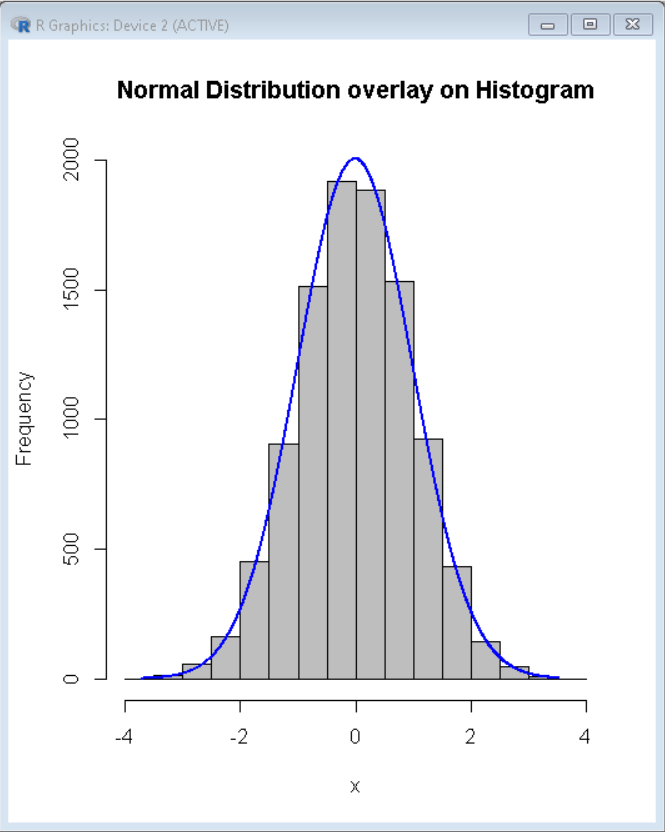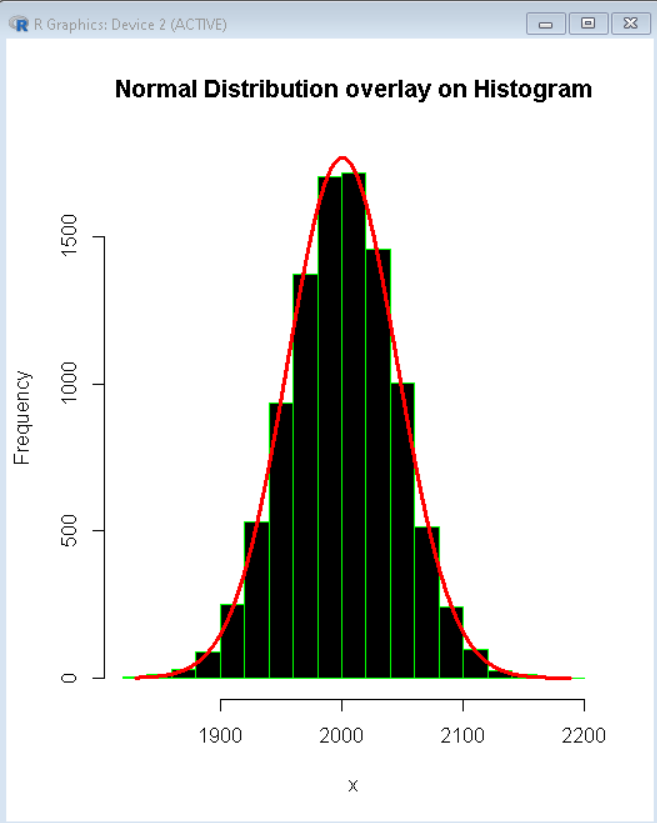Open in App
Not now

# Plot Normal Distribution over Histogram in R

• Last Updated : 28 Nov, 2021

In this article, we will discuss how to plot normal distribution over Histogram in the R Programming Language.

In a random dataset, it is generally observed that the distribution of data is normal i.e. on its visualization using density plot with the value of the variable in the x-axis and y-axis we get a bell shape curve. The center of that curve represents the mean of the data set. But the density plot even though is smooth does not show the real values but shows the normal distribution. To tackle that situation we use a histogram with a normal distribution overlay. In this scenario, the histogram gives the real values of plotted bars and the overlay density plot shows normal distribution trends.

To do so we use the plotNormalHistogram() function of the rcompanion package. This function takes the data vector as an argument and plots a histogram with the best fit density plot as an overlay.

## Installation

To install the rcompanion package:

`install.packages("rcompanion") `

## Histogram with Normal Distribution Overlay

To create a basic histogram with a normal distribution overlay of density plot, we use the plotNormalHistogram() function of the rcompanion package library in the R Language.

Syntax:

plotNormalHistogram( x, prob, col, main, length)

Parameters:

• x: Determines data vector for plot
• prob: It is a boolean value. If FALSE, then counts are displayed otherwise the density is shown.
• main: Determines the display title for the plot.
• length: Determines the number of points in the density plot line.

### Example:

Here, is a basic histogram with a normal distribution overlay.

## R

 `# load library rcompanion``library``(rcompanion)`` ` `# create data vector``x= ``c``(``rnorm``(10000))`` ` `# draw plot``plotNormalHistogram``( x, prob = ``FALSE``,``                      ``main = ``"Normal Distribution overlay on Histogram"``,``                      ``length = 1000 )`

Output:## Color and size customization

We can customize the look and feel of the above plot according to our needs by using the col, linecol, lwd, and border arguments to change the color of bars of the histogram, the color of the density plot line, width of the density plot line, and the color of the border of bars of the histogram respectively. Since, plotNormalHistogram() function is built over hist() function all arguments of hist() function also work here.

Syntax:

plotNormalHistogram( x, col, linecol, lwd, border )

Parameters

• col: Determines the color of bars of the histogram.
• linecol: Determines the color of the density plot line.
• lwd: Determines the width of the line of the density plot.
• border: Determines the color of the border of bars of the histogram.

### Example:

Here, is a histogram with normal distribution overlay with black bars with a green border and red thick density plotline.

## R

 `# load library rcompanion``library``(rcompanion)`` ` `# create data vector``x= ``c``(``rnorm``(10000, 2000, 45))`` ` `# draw plot using plotNormalHistogram() function``# use colour and size arguments for formatting plot``plotNormalHistogram``( x, prob = ``FALSE``, col=``"black"``, border=``"green"``,``                      ``main = ``"Normal Distribution overlay on Histogram"``,``                      ``length = 10000, linecol=``"red"``, lwd=3 )`

Output:My Personal Notes arrow_drop_up